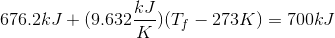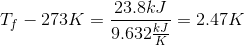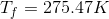# AP Physics B : Thermodynamics and Heat

## Example Questions

### Example Question #1 : Ap Physics 2

Which of the following is not an acceptable unit for specific heat?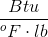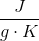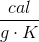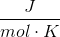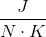Explanation:

Specific heat is most commonly applied in the equation to determine the heat required/released during a temperature change: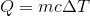Rearranging this equal, we can see that this units of specific heat are units of heat per units of mass times units of temperature.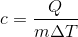Heat energy can have the units of Joules or calories. Mass can have units of grams or kilograms. Temperature can have units of Kelvin, degrees Celsius, or degrees Fahrenheit. British thermal units (Btu) is a less common unit of heat, defined by the amount of heat required to raise one pound of water by one degree Fahrenheit.

Newtons are a unit of force, and cannot be used in the units for specific heat.

### Example Question #1 : Understanding Heat And Temperature

A sample of copper is heated with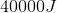of heat and the temperature of the sample rises from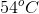to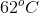. What mass of copper is present in this sample?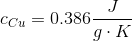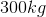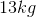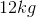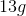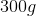Explanation:

Our equation for temperature change using specific heat is: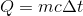Since Celsius and Kelvin use the same interval scale, we do not need to convert to find the change in temperature. Using the initial and final temperatures, the energy input, and the specific heat of copper, we can calculate the mass of copper present.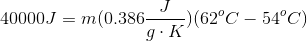To simplify, we will solve for the temperature change separately.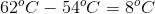Since the scale for Celsius and Kelvin is the same, this change is the same in either scale.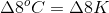Use this value back in the original calculation.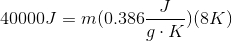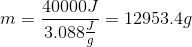Convert to kilograms to simplify.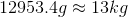### Example Question #3 : Thermodynamics And Heat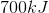of heat energy is applied to a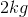block of ice initially at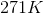. This is enough to change the ice completely to water. What is the final temperature of the water after theof heat is applied?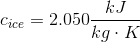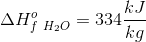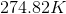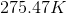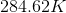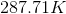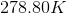Explanation:

Since the ice melts, this action occurs in three stages.

1) The heating of the ice to its melting point

2) The melting of the ice into water

3) The heating of the water after it is melted

When a temperature change is involved, the relationship of heat input to temperature change is given by:In this formula,is the specific heat (capacity) for the substance. For the phase change involved in melting, there is no temperature change (the energy goes into breaking bonds), and the amount of heat required to completely melt the substance is: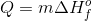So this situation is encompassed by three expressions, representing the three parts of the problem, each of which deals with the same mass of material,. Keep in mind that the melting point for ice is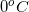, or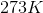.

1)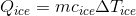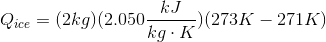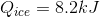2)(for the ice completely melting)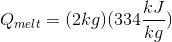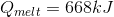3)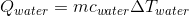(for the water warming up to its final temperature)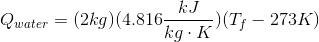The values for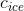,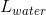, and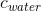are given, and it is known that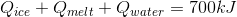.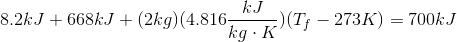Using this equation we can solve for the final temperature.